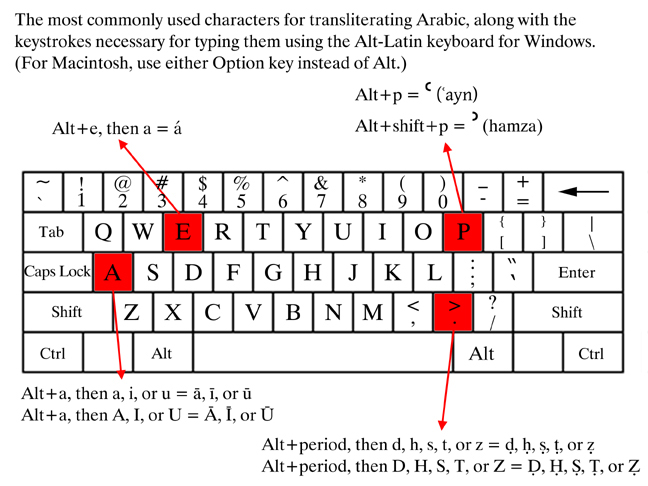# Emulating Alt-Latin keyboard layout for Arabic transcription in Vim

January 3, 2017
Tags: transcription vim
Length: short

I’m used to the Alt-Latin keyboard layout to quickly type Arabic transcription. The Alt-Latin is an extension of the US QUARTY layout that uses combinations of the ALT and SHIFT keys to add diacritics such as in and ā, as well as the characters ʿ and ʾ. A nice graphical presentation of this layout and instructions of how to install it on Windows and Mac can be found here. The image below is from that site.Below is block code I have in my `.vimrc` to emulate the Alt-Latin layout in Vim. I have only mapped characters that are needed for the transcription system used in Encyclopedia of Arabic Language and Linguistics. The code also includes mappings for `r` operations.

It may tricky to use the `r` operation with the transcription. After typing `r` to replace a single character you only have one second (with the default settings) to type the right key combination for the transcription character. If this is a problem you can change the default 1000 ms timer to some other value, e.g. `:set timeoutlen=2000`.

``````" Arabic transcription (simulates Alt-Latin keyboard layout)
inoremap <M-a>a ā
inoremap <M-a>A Ā
inoremap <M-a>i ī
inoremap <M-a>I Ī
inoremap <M-a>u ū
inoremap <M-a>U Ū
inoremap <M-a>ō ō
inoremap <M-a>Ō Ō
inoremap <M-a>e ē
inoremap <M-a>E Ē
inoremap <M-p> ʿ
inoremap <M-P> ʾ
inoremap <M-.>d ḍ
inoremap <M-.>D Ḍ
inoremap <M-.>s ṣ
inoremap <M-.>S Ṣ
inoremap <M-.>t ṭ
inoremap <M-.>T Ṭ
inoremap <M-.>z ẓ
inoremap <M-.>Z Ẓ
inoremap <M-.>h ḥ
inoremap <M-.>H Ḥ
inoremap <M-w>g ġ
inoremap <M-w>G Ġ
inoremap <M-x>d ḏ
inoremap <M-x>D Ḏ
inoremap <M-x>t ṯ
inoremap <M-x>T Ṯ
inoremap <M-v>s š
inoremap <M-v>S Š

" r operations
nmap r<M-a>a rā
nmap r<M-a>A rĀ
nmap r<M-a>i rī
nmap r<M-a>I rĪ
nmap r<M-a>u rū
nmap r<M-a>U rŪ
nmap r<M-a>ō rō
nmap r<M-a>Ō rŌ
nmap r<M-a>e rē
nmap r<M-a>E rĒ
nmap r<M-p> rʿ
nmap r<M-P> rʾ
nmap r<M-.>d rḍ
nmap r<M-.>D rḌ
nmap r<M-.>s rṣ
nmap r<M-.>S rṢ
nmap r<M-.>t rṭ
nmap r<M-.>T rṬ
nmap r<M-.>z rẓ
nmap r<M-.>Z rẒ
nmap r<M-.>h rḥ
nmap r<M-.>H rḤ
nmap r<M-w>g rġ
nmap r<M-w>G rĠ
nmap r<M-x>d rḏ
nmap r<M-x>D rḎ
nmap r<M-x>t rṯ
nmap r<M-x>T rṮ
nmap r<M-v>s rš
nmap r<M-v>S rŠ
``````